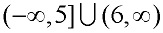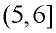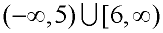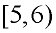Practice with Compound Inequalities Terms of Use    Contact Person: Donna RobertsDirections: Solve the following problems relating to compound inequalities. Be careful! The shout outs may, or may not, be correct.

1.
Which of the following choices is a solution to
x < -5 or x > 7 ?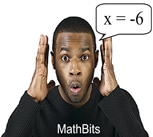Choose:
 x = -1 x = -6 x = 0 x = -4

2.
Which of the following choices is not a solution to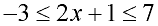?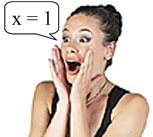Choose:
 x = 1 x = -2 x = 3 x = -4

3.
Which of the following graphs represents the solution set for: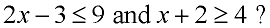Choose: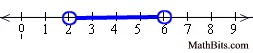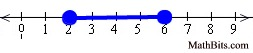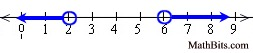4.
Which of the following graphs represents the solution set for: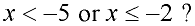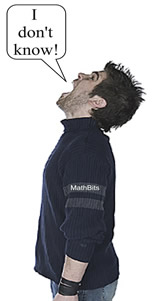Choose: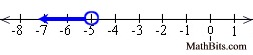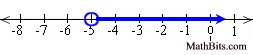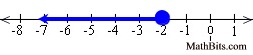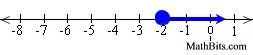5.
Which of the following choices is a solution to: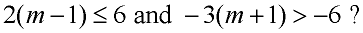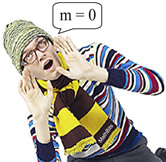Choose:
 m = 0 m = 2 m = 3 m = 4

6.
Which expressions is represented by this graph?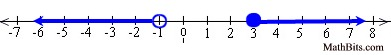Choose: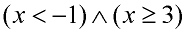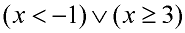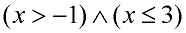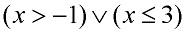7.
Which expression has the entire number line as its graph?Choose: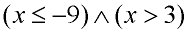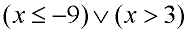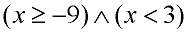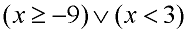8.
Which choice is the solution set for: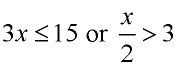Choose: